# Hard time finding the angle

• Engineering

Homework Statement
Write the two equations of equilibrium (Do not solve)
Relevant Equations
((Newton's second law))
I can't find the angle between F(Big arrow in my drawing) and the y-axis!

I am terrible in geometry, what book do you suggest to me as Mechanical Engineer to get better at geometry??

This is my attempt to solution and the figure: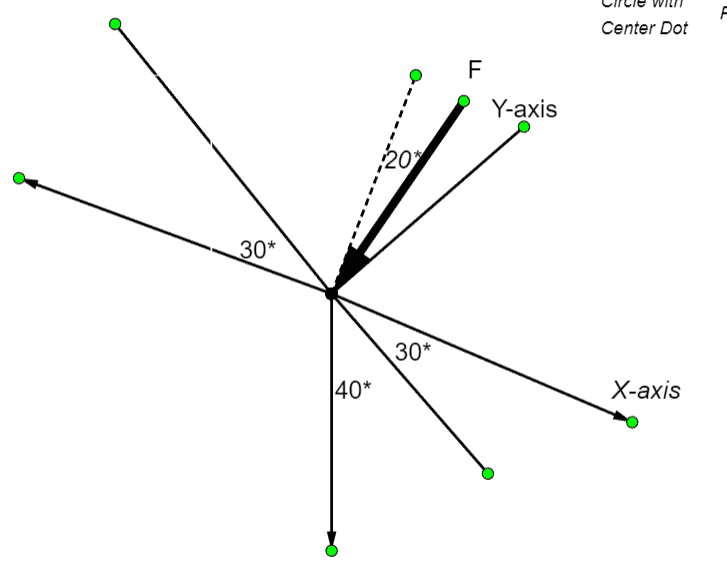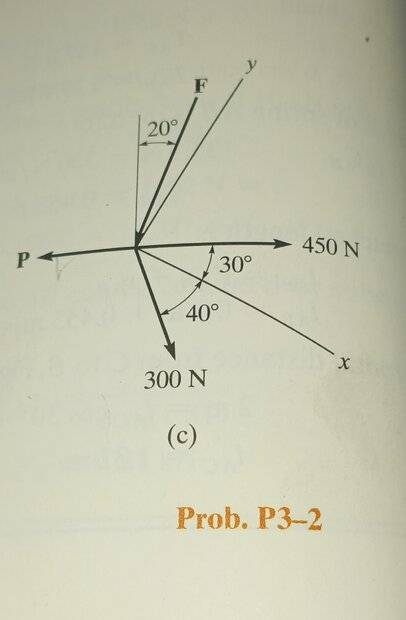Are the x and y axes supposed to be orthogonal in that drawing? And what is that other axis, maybe the z axis? And is orthogonal to the x and y axes? That's a very confusing diagram, IMO...

The cosine of the angle, ##\theta##, between two vectors, ##\vec F## and ##\vec Y## is ##\cos(\theta) = \frac {\vec F \cdot \vec Y}{ |\vec F||\vec Y|}##

That's a very confusing diagram, IMO
what are the green dots????

Note that your attempt to solution and the figure do not fully match.

Since you have the paper in fornt of your eyes:
Could we consider vectors P and 450 N colinear and both perpendicular to the reference line for 20 degrees?

Some words referring to some clear labels on the diagram would help.

The y and x axis are perpendicular
I think.

The cosine of the angle, ##\theta##, between two vectors, ##\vec F## and ##\vec Y## is ##\cos(\theta) = \frac {\vec F \cdot \vec Y}{ |\vec F||\vec Y|}##
Not very helpful as I dont know F

what are the green dots????
Never mind them, it's the drawing app start and end of the line

Are the x and y axes supposed to be orthogonal in that drawing? And what is that other axis, maybe the z axis? And is orthogonal to the x and y axes? That's a very confusing diagram, IMO...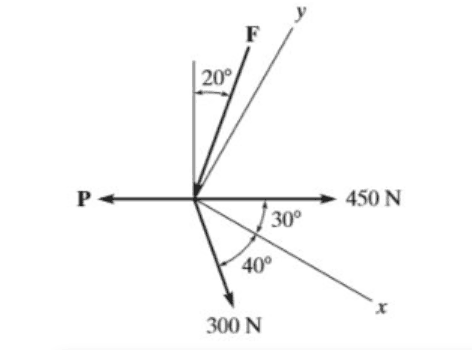This one must be better! there is zero information given in the problem

This one must be better! there is zero information given in the problem
Forget the ##x## and ##y## axes in the problem because they're included purposely to confuse you!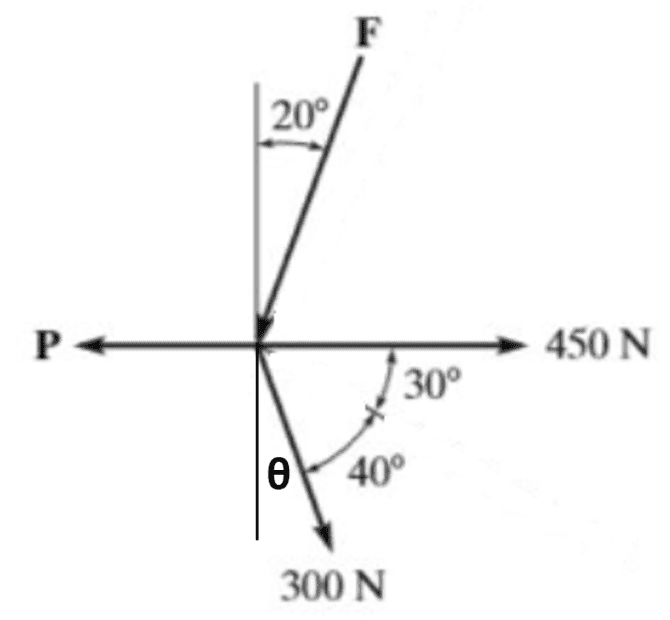Find the angle ##\theta## above and then resolve the various forces into horizontal and vertical components.

Forget the ##x## and ##y## axes in the problem because they're included purposely to confuse you!
View attachment 332183
Find the angle ##\theta## above and then resolve the various forces into horizontal and vertical components.
Okay this is helpful for other problems but this one asked me to do it using the X and Y axis!

Homework Statement: Write the two equations of equilibrium (Do not solve)
Is this the full homework statement? Where does it say anything about the ##x## and ##y##???

It only says that the sum of forces on each of the X, Y axes is zero.

It only says that the sum of forces on each of the X, Y axes is zero.
Yes, but if the sum of forces on the ##x,y## axes is zero, then those forces also sum to zero on any set of axes that are rotations of those axes, like the horizontal and vertical directions in my diagram. In general, vector forces summing to zero does not depend on any particular choice of coordinate axes: it's true for any and all of them.

•Yes, but if the sum of forces on the ##x,y## axes is zero, then those forces also sum to zero on any set of axes that are rotations of those axes, like the horizontal and vertical directions in my diagram. In general, vector forces summing to zero does not depend on any particular choice of coordinate axes: it's true for any and all of them.
Even if the new axes are not orthogonal?

Now what!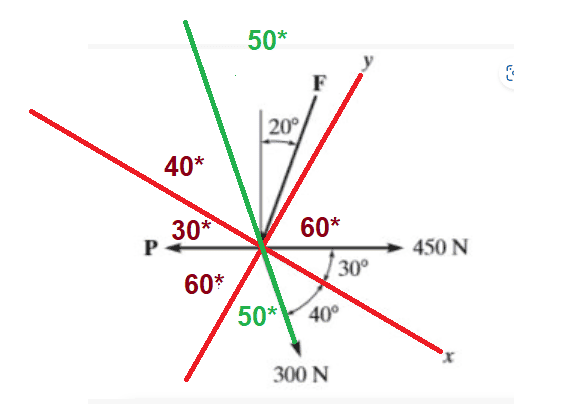Even if the new axes are not orthogonal?
Well, the horizontal and vertical axes of my diagram are a simple 20 degree rotation of the orthogonal ##x,y## axes, so they are still orthogonal axes. And yes, so long as a set of axes are not parallel to each other (i.e., the axes are a set of linearly-independent vectors), you can perfectly well resolve vectors along non-orthogonal axes to find their independent components.

•Please indulge me! Before using this busy diagram, first solve for the total horizontal and vertical forces using my diagram, set those totals to zero and write the resulting equations. (After that we can worry about finding what that solution looks like in x, y coordinates!)

Last edited:
•Please indulge me! Before using this busy diagram, first solve for the total horizontal and vertical forces using my diagram, set those totals to zero and write the resulting equations. (After that we can worry about finding what that solution looks like in ##x,y# coordinates!)
On the horizontal axis I get: 450-P+300cos70*-Fcos70*
On the Vertical axis I get: -300sin70*-Fsin70*
solving for F I get F=-300N
solving for P I get P=655N
Sorry for not using LaTeX for now.

Don't the vertical components involve the cosine of 20 degrees, not 70 degrees? And does F and P refer to the magnitudes of the force vectors F and P? If so, how can F be negative?

Don't the vertical components involve the cosine of 20 degrees, not 70 degrees? And does F and P refer to the magnitudes of the force vectors F and P? If so, how can F be negative?
No it doesn't, I read in my book that if the Magnitude came negative it means that I chose the wrong sense of direction ( need to be the opposite to fix it)
This problem is P3-2
RC.HIBBLER Statics.

No it doesn't, I read in my book that if the Magnitude came negative it means that I chose the wrong sense of direction ( need to be the opposite to fix it)
This problem is P3-2
RC.HIBBLER Statics.
Yes, and since a vector magnitude can't be negative, you need to reverse the direction of the F vector on the diagram and re-solve the problem so that the resulting F comes out positive. (Also don't forget to use the cosine of 20 degrees when you resolve the vertical components.)

•If still required, can I answer the original question...

I can't find the angle between F(Big arrow in my drawing) and the y-axisWhat is the angle between the green line and the blue vertical line?

Can you now find the angle between F and the y-axis?

Edit - severely edited as I didn’t like my first answer.

Last edited:
If still required, can I answer the original question...

View attachment 332194

What is the angle between the green line and the blue vertical line?

Can you now find the angle between F and the y-axis?

Edit - severely edited as I didn’t like my first answer.
I was very confused following your first answer the rotating and the other approach.
I still can't figure it out the angle between Vertical and Green force! I try to make equations but they cancel out!

I just can't go on in statics while I am terrible at geometry! my course starts in 18 days, what do I need to study in geometry?

I just can't go on in statics while I am terrible at geometry! my course starts in 18 days, what do I need to study in geometry?

Do not label yourself, nobody is terrible at anything.
Calm down, study and learn.

https://www.mathsisfun.com/geometry/

https://courses.lumenlearning.com/suny-physics/chapter/9-1-the-first-condition-for-equilibrium/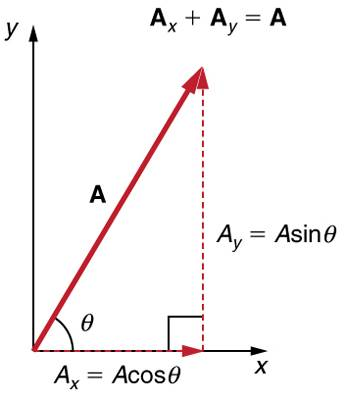Last edited:
•I just can't go on in statics while I am terrible at geometry! my course starts in 18 days, what do I need to study in geometry?

Do not label yourself, nobody is terrible at anything.
Calm down, study and learn.

Statics is all about geometry. Do lots of statics problems like this one, and you will get better and better at seeing the answers. When you get stuck on one particular problem, move on to the next ones. Then go back to the one you can't get. My experience was that the hard ones were hard because I missed seeing something that turns out to be obvious once seen. Practice practice practice.

•I was very confused following your first answer the rotating and the other approach.
I still can't figure it out the angle between Vertical and Green force! I try to make equations but they cancel out!
Sorry about the confusion. See if this helps.

Let ∠A be the angle between the blue vertical line and the Green force .

1) Force P is horizontal, so the angle between P and the vertical blue line is 90º.

2) On the diagram, this 90º angle is shown to consist of 30º, 40º and ∠A.
∠A + 30º +40º = 90º which gives ∠A = 20º.

Once you know ∠A you should be able to work out the angle between F and the y-axis.

•Sorry about the confusion. See if this helps.

Let ∠A be the angle between the blue vertical line and the Green force .

1) Force P is horizontal, so the angle between P and the vertical blue line is 90º.

2) On the diagram, this 90º angle is shown to consist of 30º, 40º and ∠A.
∠A + 30º +40º = 90º which gives ∠A = 20º.

Once you know ∠A you should be able to work out the angle between F and the y-axis.
I guessed that the Blue vertical line and the P force are perpendicular but couldn't make sure of it, that is because there is nothing to confirm it!
Now I get it.
Thanks all.

•Lnewqban and Steve4Physics
I guessed that the Blue vertical line and the P force are perpendicular but couldn't make sure of it, that is because there is nothing to confirm it!
I made the same assumption (guess) based on the fact that the original question can't be answered otherwise.

Note, if this is work you are handing-in, it's a good idea to start your answer by stating this assumption.

••